Documentation

## 5G NR Downlink ACLR Measurement

This example shows how to measure the adjacent channel leakage power ratio (ACLR) for 5G NR test models (NR-TMs) in frequency range 1 (FR1) and FR2 using 5G Toolbox™.

### Introduction

The ACLR is the ratio of the filtered mean power centered on the assigned channel frequency to the filtered mean power centered on an adjacent channel frequency. This example performs ACLR measurements for a NR downlink waveform, as defined in TS 38.104 Section 6.6.3.

### Generate NR-TM Waveform

Use the MATLAB class hNRReferenceWaveformGenerator to generate 5G NR-TMs for FR1 and FR2. You can generate the NR-TM waveforms by specifying these parameters:

• NR-TM name

• Channel bandwidth

• Subcarrier spacing

• Duplexing mode

For more information, see the 5G NR-TM and FRC Waveform Generation example.

```% Select the NR-TM waveform parameters nrtm ="NR-FR1-TM1.1"; % NR-TM name and properties bw =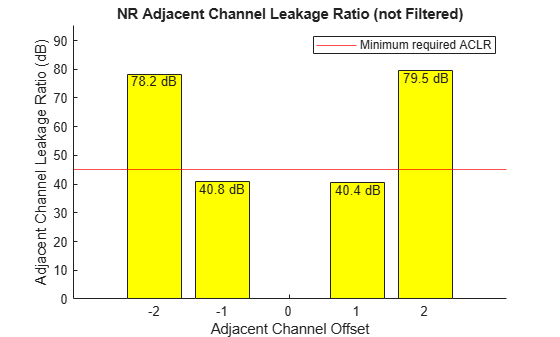"20MHz"; % Channel bandwidth scs =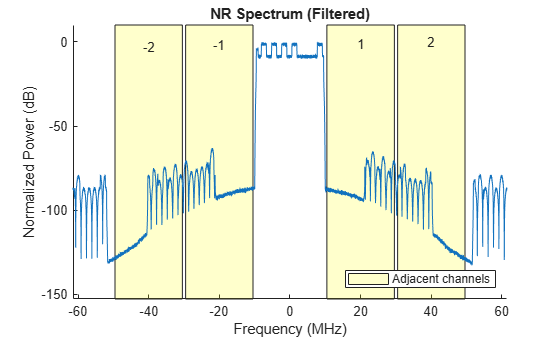"15kHz"; % Subcarrier spacing dm ="FDD"; % Duplexing mode % Create generator object for the above NR-TM tmwavegen = hNRReferenceWaveformGenerator(nrtm,bw,scs,dm); % Generate waveform [tmwaveform,tmwaveinfo] = generateWaveform(tmwavegen); samplingrate = tmwaveinfo.Info.SamplingRate; % Waveform sampling rate (Hz) % Visualize the associated PRB and subcarrier resource grids displayResourceGrid(tmwavegen);```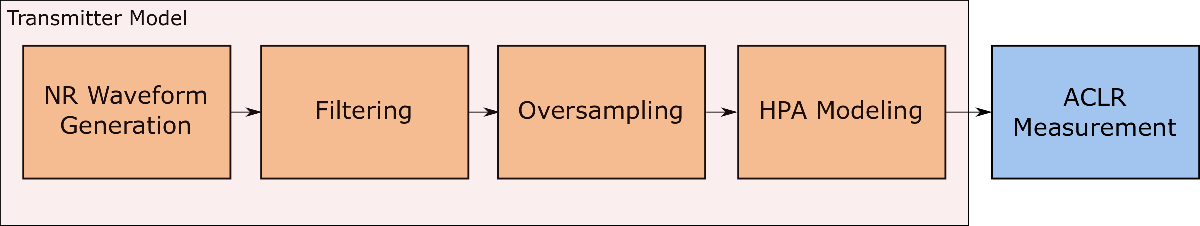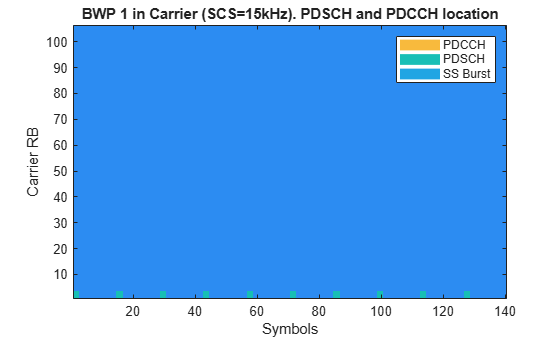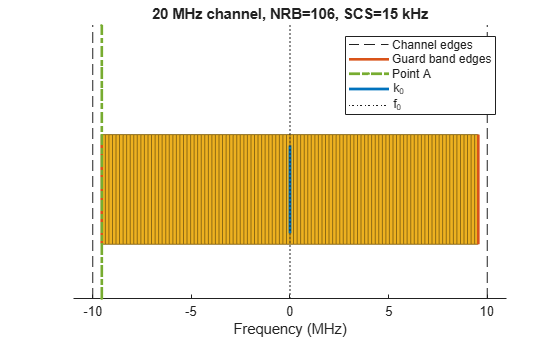### Calculate ACLR Parameters

The helper function hACLRParametersNR.m calculates the parameters required for ACLR measurement.

The function determines the required oversampling. If the input waveform sampling rate (`samplingrate`) is not sufficient to span the entire bandwidth (`aclr.BandwidthACLR`) of the adjacent channels (allowing for a maximum of 85% bandwidth occupancy), an upsampled version of the waveform is used for ACLR calculations. The upsampling factor `aclr.OSR`.

`aclr = hACLRParametersNR(tmwavegen.Config);`

### Filter Waveform to Improve ACLR

The generated waveform has no filtering, so there are significant out of band spectral emissions owing to the implicit rectangular pulse shaping in the OFDM modulation (each OFDM subcarrier has a sinc shape in the frequency domain). In order to achieve a good ACLR performance, filtering must be applied to the waveform. A filter is designed with a transition band that starts at the edge of the occupied transmission bandwidth (`aclr.BandwidthConfig`) and stops at the edge of the overall channel bandwidth (`aclr.Bandwidth`). This filter involves no rate change, it just shapes the spectrum within the original bandwidth of the waveform. The filter is first designed, then applied to the waveform.

```% Design filter lpFilt = designfilt('lowpassfir',... 'PassbandFrequency', aclr.BandwidthConfig/2,... 'StopbandFrequency', aclr.Bandwidth/2,... 'PassbandRipple', 0.1,... 'StopbandAttenuation', 80,... 'SampleRate', samplingrate); % Apply filter filtWaveform = filter(lpFilt,tmwaveform);```

### Calculate NR ACLR

The hACLRMeasurementNR.m helper function measures the NR ACLR using a square window on adjacent channels. A DFT of the measurement signal is taken and the energy of the appropriate bins used to calculate the adjacent channel powers.

```% Apply required oversampling resampled = resample(filtWaveform,aclr.OSR,1); % Calculate NR ACLR aclr = hACLRMeasurementNR(aclr,resampled);```

### Display Results

The ACLR measurement results are returned in a structure with these fields:

• `Bandwidth`: The channel bandwidth associated with `tmwaveform`, in Hertz. This is the overall bandwidth of the assigned channel.

• `BandwidthConfig`: The transmission bandwidth configuration associated with `tmwaveform`, in Hertz. This is the bandwidth within the channel bandwidth that contains active subcarriers.

• `BandwidthACLR`: The bandwidth required to represent 1st and 2nd adjacent carriers; the sampling rate used internally for ACLR measurements will support this bandwidth with at most 85% bandwidth occupancy.

• `OSR`: The integer oversampling ratio of the input `waveform` required to create a signal capable of representing 1st and 2nd adjacent carriers i.e. to represent `aclr.BandwidthACLR` with at most 85% bandwidth occupancy.

• `SamplingRate`: The sampling rate of the internal measurement signal from which the ACLR is calculated. If `OSR=1`, this signal is the input waveform; if `OSR>1`, this signal is the input waveform upsampled by `OSR`. Therefore: `aclr.SamplingRate = OSR*samplingrate`.

• `SignalPowerdBm`: The power, in decibels relative to 1mW in 1ohm, of the input within the NR channel of interest i.e. in a square filter of bandwidth `aclr.BandwidthConfig` centered at 0Hz.

• `ACLRdB`: A vector of NR ACLRs, in decibels relative to `aclr.SignalPowerdBm`, measured for adjacent channels [-2, -1, 1, 2].

• `CarrierFrequency`: A vector of NR center frequencies, in Hertz, for adjacent channels [-2, -1, 1, 2].

The helper function hACLRResultsNR.m displays the NR ACLR and plots the adjacent channel powers.

`hACLRResultsNR(aclr);`
``` Bandwidth: 20000000 SubcarrierSpacing: 15000 BandwidthConfig: 19080000 BandwidthACLR: 100000000 OSR: 4 SamplingRate: 122880000 CarrierFrequency: [-40000000 -20000000 20000000 40000000] SignalPowerdBm: -5.1358 ACLRdB: [78.5721 72.1461 72.0420 78.5704] ```### Appendix

This example uses the following helper functions and classes:

 3GPP TS 38.104. “NR; Base Station (BS) radio transmission and reception.” 3rd Generation Partnership Project; Technical Specification Group Radio Access Network.